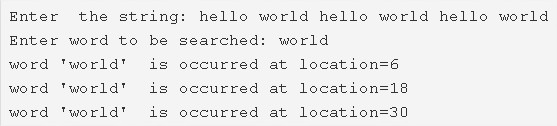# C Program To Search All Occurrences Of A Word In String | C Programs

C program to search all occurrences of a word in a given string – In this article, we will particularise the different ways to search all occurrences of a word in a given string in C programming.

Suitable examples and sample programs have also been added so that you can understand the whole thing very clearly. The compiler has also been added with which you can execute it yourself.

The ways used in this specific piece are as follows:

• Using Standard Method
• Using Function

A string is nothing but an array of characters. The value of a string is determined by the terminating character. Its value is considered to be 0.The image uploaded above states that first, you need to enter the string concerned.

The string entered here is as follows:

“hello world hello world hello world”

In the next step, you need to specify what is the word that you want to be searched.

The word “world” is listed for the search part.

Thus, it is seen that the word “world” is spotted at locations 6, 18 and 30.

Thus, the numerous means to do so in C programming are as follows:

## Using Standard Method

1. The function gets(s) reads the entered string and it stores the string in the character arrays[].

2) The gets(w) function reads the entered word and saves in the character array w[].

3) For loop iterates through the string and finds the index values of the white spaces, stores the index values in the integer array a[].

4) The for loop iterates through the white spaces of the string with the structure for(i=0; i<k;i++)

a) Initialize n=a[i]-j i.e first white space index-j

b) If the length of the word is equal to n

b.1) Then t=0, compare the first element of the word with the first element of the string as s[l+j]==w[l]. If matched then increase the ‘t’ value. Repeat this for all elements of the word by increasing l value. If t=length of the word then increase the ‘found’ value by 1 and print the location of the occurrence of the word in the string i.e. j.

If the given word has not occurred before the first whitespace then initialize j=a[i]+1 and go to the next index of the whitespace by increasing i value. Repeat until i<k.

Output:

### Using Function

1. The main() call the check(char *s, char *w) function to find all occurrences of the word in the given string.

2) The function

a) Iterates through the string using for loop(i=0;s[i];i++) and finds the index values of the white spaces and place the index values in the integer array a[].

b) j=0, Iterate through the white spaces until i<k,

Initialize n=a[i]-j

b.1) If the length of the word is equal to the first index value of white space then compare each letter of the word with the elements of the string as s[i+l]==w[l] by increasing l value. If matched then t++. If ‘t’ is equal to the length of the word then print the location of occurrence of the word.

If the word has not occurred before the first whitespace then initialize j=a[i]+1 and go for next index of the white space. Repeat the loop until i<k.

Output:

x

## C Program To Print Number Of Days In A Month | Java Tutoring

C program to input the month number and print the number of days in that ...